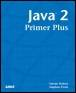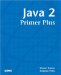# Understanding Object ReferencesJava™ 2 Primer PlusBy Steven Haines, Steve Potts Table of Contents Chapter 6.  Classes

When you create a variable that is an instance of a class, you are actually creating a reference to that class instance. The reference is defined to know how to access its class, but not to be tightly coupled with any particular instance. If you were to create two instances of a class, and then assign one to the other, both variables will be referencing the same object. Listing 6.7 demonstrates this.

##### Listing 6.9 Number.java
` public class Number {     private int number;     public Number( int number ) {        this.number = number;     }     public int getNumber() {        return this.number;     }     public void setNumber( int number ) {        this.number = number;     }     public static void main( String[] args ) {        Number one = new Number( 1 );        Number two = new Number( 2 );        System.out.println( "Beginning: " );        System.out.println( "One = " + one.getNumber() );        System.out.println( "Two = " + two.getNumber() );        // Assign two to one        two = one;        System.out.println( "\nAfter assigning two to one: " );        System.out.println( "One = " + one.getNumber() );        System.out.println( "Two = " + two.getNumber() );        // Change the value of two        two.setNumber( 3 );        System.out.println( "\nAfter modifying two: " );        System.out.println( "One = " + one.getNumber() );        System.out.println( "Two = " + two.getNumber() );     }  } `

The output of the Number class is

` Beginning:   One = 1  Two = 2   After assigning two to one:  One = 1  Two = 1  After modifying two:  One = 3  Two = 3 `

From this output you can see that assigning the Number variable two to the Number variable one actually made two point to the same memory as one. Then, when two was modified, it affected the value of one.

Therefore, when you pass an object reference to a method, it is passed to that method by reference. If the method modifies the object, the original object is also modified. This is a powerful feature for performance because the Java Runtime Engine does not have to make a copy of the class when passing it to a method, but be aware of the potential side effects!TopJava 2 Primer Plus
ISBN: 0672324156
EAN: 2147483647
Year: 2001
Pages: 332

Similar book on Amazon